• +91 9971497814
• info@interviewmaterial.com

# Polynomials Ex-2.2 Interview Questions Answers

### Related Subjects

Question 1 :

Find the zeroes of the following quadraticpolynomials and verify the relationship between the zeroes and thecoefficients.

(i) x2–2x –8

Solutions:

(i) x2–2x –8

x2– 4x+2x–8

= x(x–4)+2(x–4)

= (x-4)(x+2)

Therefore, zeroes of polynomial equation x2–2x–8are (4, -2)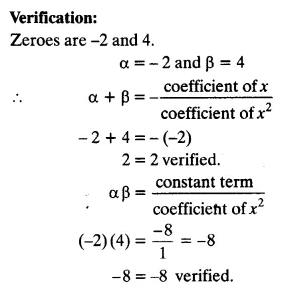(ii) 4s2–4s+1

Solutions:

(ii) 4s2–4s+1

4s2–2s–2s+1

=2s(2s–1)–1(2s-1)

= (2s–1)(2s–1)

Therefore, zeroes of polynomial equation 4s2–4s+1are (1/2, 1/2)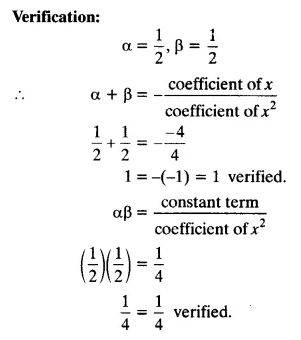(iii) 6x2–3–7x

Solutions:

(iii)6x2–3–7x

6x2–7x–3

= 6x–9x + 2x – 3

= 3x(2x – 3) +1(2x – 3)

= (3x+1)(2x-3)

Therefore, zeroes of polynomial equation 6x2–3–7xare (-1/3, 3/2)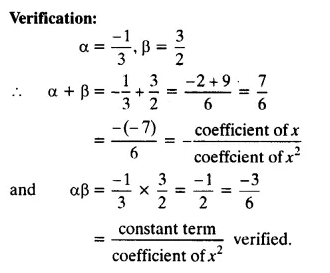(iv) 4u2+8u

Solutions:

(iv) 4u2+8u

4u(u+2)

Therefore, zeroes of polynomial equation 4u2 +8u are (0, -2).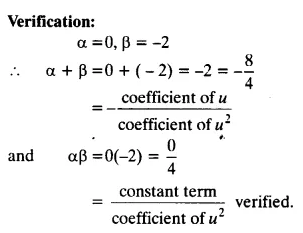(v) t2–15

Solutions:

(v) t2–15

t2 = 15 or t = ±√15

Therefore, zeroes of polynomial equation t2 –15are (√15, -√15)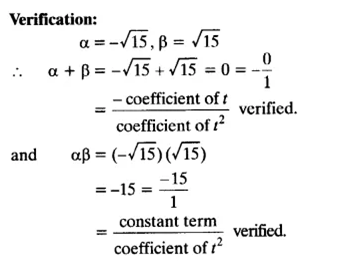(vi)   3x2–x–4

Solutions:

(vi)  3x2–x–4

=3x2–4x+3x–4

= x(3x-4)+1(3x-4)

= (3x – 4)(x + 1)

Therefore, zeroes of polynomial equation3x2 –x – 4 are (4/3, -1)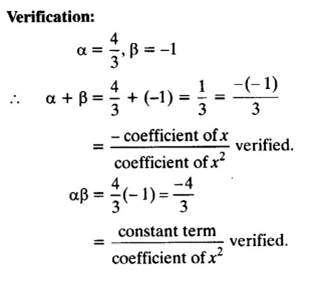Question 2 :

Find a quadratic polynomial each with thegiven numbers as the sum and product of its zeroes respectively.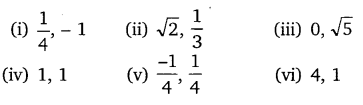(i) 1/4 , -1

Solution:

Let the zeroes of polynomial be α and β.

Now, Sum of zeroes = α+β = 1/4

Product of zeroes = α β = -1

If α and β are zeroes of any quadratic polynomial, then thequadratic polynomial equation can be written directly as:-

x2–(α+β)x +αβ = 0

x2–(1/4)x +(-1) = 0

4x2–x-4 = 0

(ii)√2, 1/3

Solution:

Let the zeroes of polynomial be α and β.

Sum of zeroes = α + β =√2

Product of zeroes = α β = 1/3

If α and β are zeroes of any quadratic polynomial, then thequadratic polynomial equation can be written directly as:-

x2–(α+β)x +αβ = 0

x2 –(√2)x + (1/3) = 0

3x2-3√2x+1 = 0

Thus, 3x2-3√2x+1 is the quadratic polynomial.

(iii) 0, √5

Solution:

Let the zeroes of polynomial be α and β.

Sum of zeroes = α+β = 0

Product of zeroes = α β = √5

If α and β are zeroes of any quadratic polynomial, then thequadratic polynomial equation can be written directly

as:-

x2–(α+β)x +αβ = 0

x2–(0)x +√5= 0

Thus, x2+√5 is the quadratic polynomial.

(iv) 1, 1

Solution:

Let the zeroes of polynomial be α and β.

Sum of zeroes = α+β = 1

Product of zeroes = α β = 1

If α and β are zeroes of any quadratic polynomial, then thequadratic polynomial equation can be written directly as:-

x2–(α+β)x +αβ = 0

x2–x+1 = 0

Thus , x2–x+1is the quadratic polynomial.

(v) -1/4, 1/4

Solution:

Let the zeroes of polynomial be α and β.

Sum of zeroes = α+β = -1/4

Product of zeroes = α β = 1/4

If α and β are zeroes of any quadratic polynomial, then thequadratic polynomial equation can be written directly as:-

x2–(α+β)x +αβ = 0

x2–(-1/4)x +(1/4) = 0

4x2+x+1 = 0

(vi) 4, 1

Solution:

Let the zeroes of polynomial be α and β.

Sum of zeroes = α+β =

Product of zeroes = αβ = 1

If α and β are zeroes of any quadratic polynomial, then thequadratic polynomial equation can be written directly as:-

x2–(α+β)x+αβ = 0

x2–4x+1 = 0

Thus, x2–4x+1 is the quadratic polynomial.

Todays Deals### Polynomials Ex-2.2 Contributorskrishan

Name:
Email:

# Latest News# 9000 interview questions in different categories